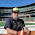### Physics 9B Practice Final Problem #5

Two volumes A and B of ideal gas with NA and NB molecules respectively are separated by a piston that is free to move while the total volume V = VA + VB is constant. Both gases are in contact with a heat reservoir at a fixed temperature T.
For each volumes of gas A and B, the multiplicity depends on the volume according to
ωA = aVANA
ωB = bVBNB
with a and b independent of VA and VB . After the piston comes to equilibrium, what is the pressure in the gas? Express it in terms of k, V, NA, NB, T, a, and b. Do the problem using the given information and the ideas of statistical mechanics. There are at least a couple ways to do the problem. If you want to use the equation of state, you must derive it from the information given above and general results on the properties of entropy.
Question so kindly provided by Joe Kiskis

Edit: Simply put, this is all wrong. It doesn't even help you, so ignore it.
Like all of the statistical mechanics problems for this class, the proofs just feel like I'm making stuff up until the needed variable happens to be by itself on one side.

For this one, we're going to use:
• S=k ln(ω)
• ∂S⁄∂V|U = P⁄T
Beginning with S=k ln(ω):
1. S = k ln[abVANAVBNB]
• Substitute in the equations for ω
2. S = k ln[ab(V−VB)NA(V−VA)NB]
• Put in terms of V
3. ∂S⁄∂V = abk [NA(V−VB)NA−1(V−VA)NB + NB(V−VA)NB−1(V−VB)NA] ⁄ [ab(V-VB)NA (V−VA)NB]
• Take partial with respect to V
4. ∂S⁄∂V = k[NA(V−VA)+NB(V−VB)]⁄[(V−VB)(V−VA)]
• Just simplified
5. P⁄T = k[NA(V−VA)+NB(V−VB)]⁄[(V−VB)(V−VA)]
• Substitute in ∂S⁄∂V|U = P⁄T, assuming that we're at equilibrium so U really is constant.
6. P = kT[NA(V−VA)+NB(V−VB)]⁄[(V−VB)(V−VA)]
• Solve for P
Do I think its correct? God no… I do think it's a pretty fair argument, which very well might get me some of the points…

Note: The correct answer is P = kT(NA+NB)⁄V, which you can totally see in my answer, but I don't know how to get there from here.

1.Well, on the chatroom, the Professor show us the answer "P=kT(N_A+N_B)/V ". Does that mean your answer is right? I didn't really understand this question to begin with so please bare with me.

2.No, my answer is wrong, but it's very close.

3.is there a way we can make N_a and N_b = 0. cuz then that's the answer.

4.Could substituting V_a = V - V_b or V_b = V - V_a help if you substitute it to your final equation?

5.Making N_A or N_B = 0 is nonsense. You can't set the number of molecules to zero.

As for the other idea, I don't see it being useful. I'm going to go to the study session and see what we can come up with as a group.

6.oops, I meant V_a and V_b not N_a or N_b, but i guess it doesn't make a lot of sense to make the volume zero either...